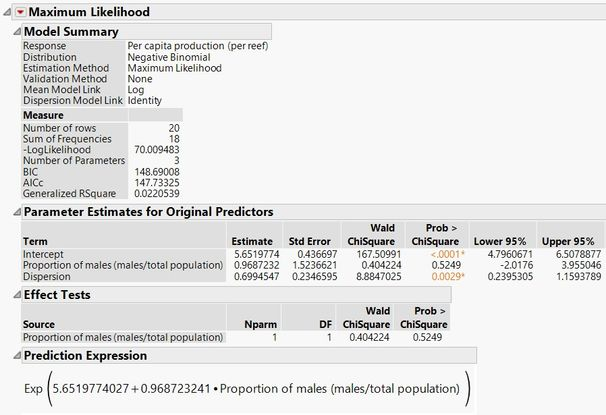Our World Statistics Day conversations have been a great reminder of how much statistics can inform our lives. Do you have an example of how statistics has made a difference in your life? Share your story with the Community!
Choose Language Hide Translation Bar
Highlighted

## How to perform a negative binomial regression in JMP?

Hi,

I was wondering how to perform a negative binomial regression in JMP. I had previously done a Poisson regression using the Generalized Linear Model but am experiencing overdispersion with my data.

Thank you.

1 ACCEPTED SOLUTION

Accepted Solutions
Highlighted

## Re: How to perform a negative binomial regression in JMP?

With JMP Pro, you specify Fit Model. change the Personality to Generalized Regression and the Distribution to Negative Binomial.

Dan Obermiller
6 REPLIES 6
Highlighted

## Re: How to perform a negative binomial regression in JMP?

With JMP Pro, you specify Fit Model. change the Personality to Generalized Regression and the Distribution to Negative Binomial.

Dan Obermiller
Highlighted

## Re: How to perform a negative binomial regression in JMP?

Great, thanks for the quick reply.

Quick question, what's the difference between doing a Poisson regression under the Generalized Regression personality as opposed to a Poisson regression under the General Linear Model personality?

Edit: Ran a Poisson and Negative Binomial regression under the Generalized Regression personality. I'm a bit unfamiliar with the output. Are there any guides/walk-throughs that can help me interpret what I'm seeing? Thank you.

Highlighted

## Re: How to perform a negative binomial regression in JMP?

They can be exactly the same. With Generalized Regression, you can choose Poisson with Maximum Likelihood estimation and the results will match the Generalized Linear Models Poisson regression with a Log Link function.

With Generalized Regression you have different estimation methods such as  Maximum Likelihood, Lasso, Elastic Net, Ridge, Double Lasso, etc. With Generalized Linear Models you have only Maximum Likelihood Estimation. Also, with Generalized Regression you have a much greater choice of distributions to pick from.

Dan Obermiller
Highlighted

## Re: How to perform a negative binomial regression in JMP?

There is a full class called Finding Important Predictors that shows many of the details behind Generalized Regression. Details can be found on the JMP Training site https://support.sas.com/edu/schedules.html?ctry=us&crs=JFIP.

For some more immediate on-demand videos, you can find a few on www.jmp.com. Here is one that might prove helpful: https://www.jmp.com/en_us/events/ondemand/mastering-jmp/generalized-regression.html. There are a few others that you can probably find in that location as well.

Dan Obermiller
Highlighted

## Re: How to perform a negative binomial regression in JMP?

Thanks. That did help.

A quick question, I was wondering if you could help me interpret the output from this analyses. It's a simple negative binomial regression with only one predictor variable.I'm assuming that treatment is not significant (p = 0.525) and only explained 2.2% of the variability within the model. Is this correct? Furthermore, how should I interpret the significance of the dispersion parameter?

I apologize for all of the questions, and I really appreciate your patience and input.

Highlighted

## Re: How to perform a negative binomial regression in JMP?

Hi, I would be very grateful if you could please advise on the following. I run JMP 13 (not the pro edition since I have a student licence) and I would like to run a negative binomial model. It seems under the distribution for GLM I can only find (normal, binomial, poisson and exponential) - is there any option somewhere else to run a negative binomial rather than binomial or poisson? many thanks!

Article Labels

There are no labels assigned to this post.